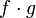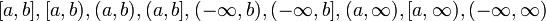Continuous functions form a unital algebra

Statement

Continuity at a point version

Suppose$c \in \R$. Then, the following are true:

• Additive closure: If$f,g$ are functions defined in open intervals containing$c$ and both of them are continuous at$c$, then the pointwise sum$f + g$ is continuous at$c$.
• Scalar multiples: If$f$ is defined in an open interval containing$c$ and is continuous at$c$, and$\lambda$ is a real number, then$\lambda f$ is continuous at$c$.
• Multiplicative identity: The constant function that sends everything to$1$ is continuous at$c$.
• Multiplicative closure: If$f,g$ are functions defined in open intervals containing$c$ and both of them are continuous at$c$, then the pointwise product$f \cdot g$ is continuous at$c$.

There is a technical way of forming a unital algebra (a vector space endowed with an identity and a compatible multiplication) from the set of continuous functions at the point$c$, once we identify (as being the same) any two functoins that agree on an open interval containing$c$. This approach is called taking the germ of the function.

Continuity around a point version

Suppose$c \in \R$. Then, the following are true:

• Additive closure: If$f,g$ are functions defined and continuous in open intervals containing$c$, then the pointwise sum$f + g$ is continuous at$c$.
• Scalar multiples: If$f$ is defined and continuous in an open interval containing$c$>, and$\lambda$ is a real number, then$\lambda f$ is continuous at$c$.
• Multiplicative identity: The constant function that sends everything to$1$ is continuous in an open interval containing$c$.
• Multiplicative closure: If$f,g$ are functions defined and continuous in open intervals containing$c$, then the pointwise product$f \cdot g$ is continuous at$c$.

There is a technical way of forming a unital algebra (a vector space endowed with an identity and a compatible multiplication) from the set of continuous functions at the point$c$, once we identify (as being the same) any two functoins that agree on an open interval containing$c$. This approach is called taking the germ of the function.

Continuity on an interval version

Suppose$I$ is an interval (possibly open, closed, or infinite from the left side and possibly open, closed, or infinite from the right side -- so it could be of the form$[a,b],[a,b),(a,b),(a,b],(-\infty,b),(-\infty,b],(a,\infty),[a,\infty),(-\infty,\infty)$). A continuous function on$I$ is a function on$I$ that is continuous at all points on the interior of$I$ and has the appropriate one-sided continuity at the boundary points (if they exist).

The continuous functions on$I$ form a real vector space, in the sense that the following hold:

• Additive: A sum of continuous functions is continuous: If$f,g$ are both continuous functions on$I$, so is the pointwise sum of functions$f + g$.
• Scalar multiples: If$\lambda \in \R$ and$f$ is a continuous function on$I$, then$\lambda f$ is also a continuous function on$I$.
• Multiplicative identity': The constant function sending everything to 1 is a continuous function on$I$.
• Multiplicative closure: A product of continuous functions is continuous: If$f,g$ are both continuous functions on$I$, so is the pointwise product of functions$f \cdot g$.

Facts used

1. Continuous functions form a vector space: This takes care of the addition and scalar multiples aspects
2. Limit is multiplicative: This takes care of multiplicative closure